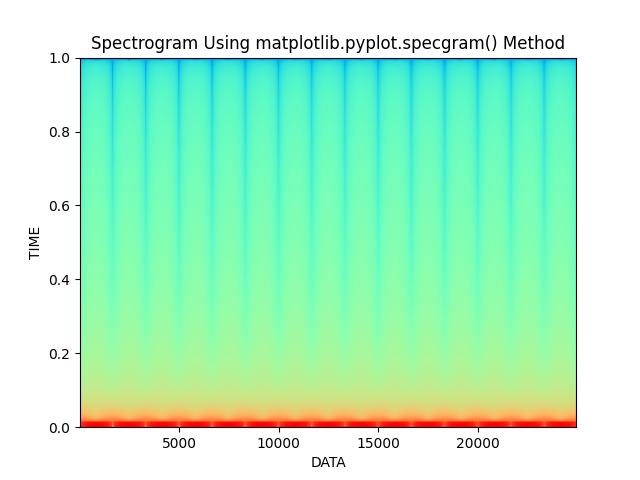# Plotting a Spectrogram using Python and Matplotlib

• Difficulty Level : Medium
• Last Updated : 19 Jan, 2022

Prerequisites: Matplotlib

A spectrogram can be defined as the visual representation of frequencies against time which shows the signal strength at a particular time. In simple words, a spectrogram is nothing but a picture of sound. It is also called voiceprint or voice grams. A spectrogram is shown using many colors which indicates the signal strengths. If the color is bright then it means that the energy of the signal is high. In other words, brightness of the color is directly proportional to the strength of the signal in spectrogram.

The spectrograms are actually created using Short-time Fourier Transform(STFT). It helps us to do a time-varying analysis of the signal provided. Anyway, it is not required to get into the depth of this topic. The main concept is that we divide the audio signal into small pieces and then that audio signal is plotted on the graph against time.

For this visualization specgram() function is used with the required parameters.

Syntax: matplotlib.pyplot.specgram(Data, NFFT=None, Fs=None, Fc=None, detrend=None, window=None, noverlap=None, cmap=None, xextent=None, pad_to=None, sides=None, scale_by_freq=None, mode=None, scale=None, vmin=None, vmax=None, *, data=None, **kwargs)

Parameter:

• Data- This is the sequence of actual data that needs to be plotted.
• Fs- This is a scaler with a default value of 2.
• window- This parameter converts the data segment and returns the windowed version of the segment.
• sides- This specifies the side of the spectrum which should be displayed. It can have three values, namely, “default”, “onesided” and “twosided”.
• NFFT- This parameter contains the number of data points used in each block for the FFT.
• detrend- This parameter contains the function applied to each segment before fitting.
• scale_by_freq- This parameter is allows for integration over the returned frequency values.
• mode- This parameter is that what sort of spectrum to use {‘default’, ‘psd’, ‘magnitude’, ‘angle’, ‘phase’}.
• noverlap- This parameter is the number of points of overlap between blocks.
• scale- This contains the scaling of the values in the spec  and can have three values as ‘default’, ‘linear’ and ‘dB’.
• Fc : This parameter is the center frequency of x.
• camp: This parameter is a matplotlib.colors.Colormap instance which allows us to change the colors of the spectrogram.

These were the basics of the spectrogram. Now, let’s move on to plotting a spectrograph using matplotlib library in python.

### Approach

• Import module
• Set the time difference to take picture of the generated signal
• Generate an array of values
• Use the function with correct parameters
• Add additional customization to the plot
• Display plot

Example:

## Python3

 `# Importing libraries using import keyword.``import` `math``import` `numpy as np``import` `matplotlib.pyplot as plt` `# Set the time difference to take picture of``# the the generated signal.``Time_difference ``=` `0.0001` `# Generating an array of values``Time_Array ``=` `np.linspace(``0``, ``5``, math.ceil(``5` `/` `Time_difference))` `# Actual data array which needs to be plot``Data ``=` `20``*``(np.sin(``3` `*` `np.pi ``*` `Time_Array))` `# Matplotlib.pyplot.specgram() function to``# generate spectrogram``plt.specgram(Data, Fs``=``6``, cmap``=``"rainbow"``)` `# Set the title of the plot, xlabel and ylabel``# and display using show() function``plt.title(``'Spectrogram Using matplotlib.pyplot.specgram() Method'``)``plt.xlabel(``"DATA"``)``plt.ylabel(``"TIME"``)``plt.show()`

Output:My Personal Notes arrow_drop_up